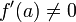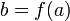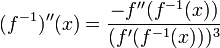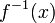# Second derivative rule for inverse function

View other differentiation rules

## Statement

### Simple version at a specific point

Suppose$f$ is a one-one function and$a$ is a point in the domain of$f$ such that$f$ is twice differentiable at$a$ and$f'(a) \ne 0$ where$f'$ denotes the derivative of$f$. Suppose$b = f(a)$.

Then, we have the following formula for the second derivative of the inverse function$f^{-1}$:$(f^{-1})''(b) = \frac{-f''(a)}{(f'(a))^3}$

### Simple version at a generic point

Suppose$f$ is a one-one function. Then, we have the following formula:$(f^{-1})''(x) = \frac{-f''(f^{-1}(x))}{(f'(f^{-1}(x)))^3}$

where the formula is applicable for all$x$ in the range of$f$ for which$f$ is twice differentiable at$f^{-1}(x)$ and the first derivative of$f$ at$f^{-1}(x)$ is nonzero.

MORE ON THE WAY THIS DEFINITION OR FACT IS PRESENTED: We first present the version that deals with a specific point (typically with a$\{ \}_0$ subscript) in the domain of the relevant functions, and then discuss the version that deals with a point that is free to move in the domain, by dropping the subscript. Why do we do this?
The purpose of the specific point version is to emphasize that the point is fixed for the duration of the definition, i.e., it does not move around while we are defining the construct or applying the fact. However, the definition or fact applies not just for a single point but for all points satisfying certain criteria, and thus we can get further interesting perspectives on it by varying the point we are considering. This is the purpose of the second, generic point version.